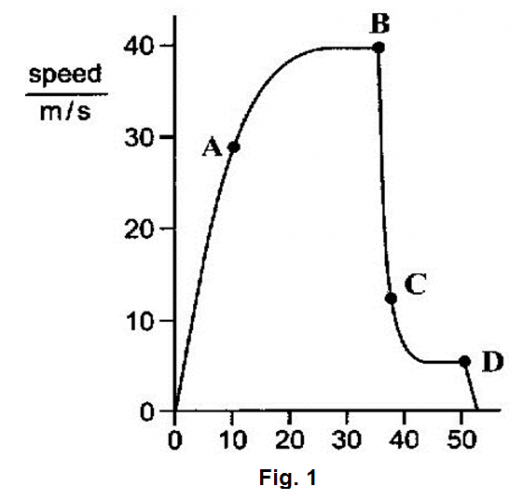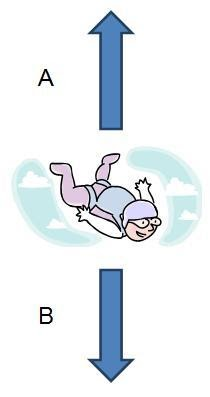# EdPlace's GCSE home learning physics lesson: Newton's 3 Laws of Motion - Forces

Looking for short lessons to keep your child engaged and learning? Our experienced team of teachers have created English, maths and science lessons for the home, so your child can learn no matter where they are.  And, as all activities are self-marked, you really can encourage your child to be an independent learner.

Get them started on the lesson below and then jump into our teacher-created activities to practice what they've learnt. We've recommended five to ensure they feel secure in their knowledge - 5-a-day helps keeps the learning loss at bay (or so we think!).

Are they keen to start practising straight away? Head to the bottom of the page to find the activities.

Now...onto the lesson!

For all GCSE Physics and Combined Science specifications, the Forces module is a substantial amount of the specification.  For example, AQA Physics (and Trilogy Science): Module 5, OCR Physics (and Combined Gateway Science): Module 1, Edexcel Physics (and Combined Science): Topic 2

Physics is often seen as the most challenging of the sciences by many students. This is often due to students finding it difficult to model the content and apply it to real-life examples. This, however, is something that students must try to do.

The topic of Forces is a large section of the GCSE physics course in all specifications.  As a parent, our top tip for supporting your child to tackle this topic is by studying how forces change during a skydive and link this to Newton’s 3 Laws of Motion. Not only will this help them learn about forces, but by applying it to this example and making them explain it back to you will help them to think more deeply and apply it to new situations.

We're confident if you follow this step by step approach your child will:

1) Understand Newton’s 3 Laws of Motion and be able to define them

2) Apply all three to different stages of a skydive

3) Explain how forces change during a skydive using a graph back to you in detail

## Step 1: Understanding the Scientific Terminology

This topic is full of understanding but relatively few examples of scientific terminology. However, below are some examples of some keywords that students must understand in order to be able to explain forces correctly.

A resultant force is the total effect of all the forces acting on an object.

Terminal Velocity is the name given when a skydiver reaches their maximum constant speed.

Velocity means speed in a given direction. Skydivers have a velocity as they are always moving at a speed downwards.

## Step 2: Prior Knowledge Required...

A force is a push or pull. It can change the speed or direction of an object (although at GCSE we only look at examples changing the speed of objects).  There are two types of forces:

Non-contact forces occur between two objects that interact without touching and include: gravity, electrostatic forces and magnetism.

Contact forces occur when two objects touch and include: friction, air resistance and tension.

Gravity is a non-contact force and has a strength of about 10 N/kg anywhere on Earth (for AQA you must learn 9.8 N / kg).

Gravity affects our weight which is a force and pulls us downwards. The equation which links gravity and weight is:

Weight = gravitational field strength x mass

Let’s use an example for the rest of this lesson.

My mass is 75 kg. If we take gravitational field strength on Earth as 10 N / kg we can calculate my weight.

Weight = gravitational field strength x mass

Weight = 10 N/kg x 75 kg

My weight is 750 N.

This weight of 750 N will not change anywhere on Earth and, therefore, my downwards force during a skydive will always be my weight at 750 N.

On a free body diagram, we represent forces with arrows. The relative sizes of the arrows show the sizes of each force acting on the object.

## Step 3: Putting this Onto a Graph...

When completing a skydive the jumper’s safety relies fully on the Laws of Motion in order to safely land. A falling object accelerates due to the downward pull of gravity. If there was no upwards force of air resistance they would accelerate constantly until they hit the ground.  Thankfully the air resistance acting as a drag force slows them at different points of the jump.

We are going to split the jump into several points as shown on the graph and for each of them we are going to answer 4 questions:

1) What is happening to the speed of the skydiver?

2) What the relative sizes are affecting the skydiver?

3) Which of Newton’s Laws of Motion does this link to?

4) What is causing these forces to be present?Point A on the graph:

At point A the skydiver is accelerating which means their velocity (their downwards speed) is increasing.

This is because the downwards force of weight is larger than the upwards force of air resistance.

This follows Newton’s Second Law of Motion which states when forces acting on an object are not balanced the speed of that object will change. In this case, the skydiver will accelerate towards the ground.

Between Point A and B on the graph:

If you follow the line on the graph it becomes horizontal which shows you the velocity (downward speed) of the skydiver becomes constant at 40 m / s.

This is because the downward force of weight becomes the same as the upwards force of air resistance and we say the resultant force is zero.

This follows Newton’s First Law of Motion which states when forces acting on an object are balanced (equal size) the speed of that object will remain constant.

The skydiver is said to have reached their terminal velocity as the upward force of air resistance stops them accelerating any further.Point B on the Graph:

At this point on the graph, the velocity (downward speed) of the skydiver suddenly drops from 40 m/s to about 12 m/s.

This is because they have opened the parachute and the upwards force of air resistance becomes much larger than the downward force of weight.

This follows Newton’s Second Law of Motion which states when forces acting on an object are not balanced the speed of that object will change. In this case, the skydiver will slow down as the upwards force of air resistance is larger making it safer for them as they get to the ground.

The upwards force of air resistance becomes larger due to opening the parachute. Parachutes have a large surface area which means more air particles collide with it as the skydiver falls and this slows them down.Point C on the graph:

If you follow the line on the graph it becomes horizontal again which shows you the velocity (downward speed) of the skydiver becomes constant at 12 m/s.

This is because the downward force of weight becomes the same as the upwards force of air resistance and we say the resultant force is zero.

This follows Newton’s First Law of Motion which states when forces acting on an object are balanced (equal size) the speed of that object will remain constant.

The skydiver is said to have reached their second terminal velocity as the upward force of air resistance has decreased back down to 750 N as they have slowed and thus the forces are balanced.

Point D on the graph:

The skydiver’s velocity quickly drops to zero as they reach the ground.

You don’t need to be able to explain this in terms of relative forces, but you need to understand that the skydiver stops because of Newton’s Third Law of Motion.

Newton’s Third Law of Motion states that when two objects interact and a force is applied by the first object onto the second object. The second object will exert an equal and opposite force back on the first object.  In this example, if the skydiver lands with a force of 750 N onto the ground. The ground will exert an equal (750 N) and opposite (upwards) force back on the skydiver.

## Step 4: Give it a go!

Why not apply the above to the following example?

1) What is happening to the skydiver’s speed in the following example and how do you know? (3 marks)2) Why does opening the parachute slow the skydiver falling speed?  (3 marks)

Stretch and challenge:

3) What is Newton’s Third Law of Motion? (2 marks)

4) What is the resultant force acting on the skydiver 30 seconds into the jump and how do you know? (3 marks).

## Step 5 - Putting it into Practise

Now, you’ve covered this together why not put this to the test and assign your child the following 5 activities in this order.

All activities are created by teachers and automatically marked. Plus, with an EdPlace subscription, we can automatically progress your child at a level that's right for them. Sending you progress reports along the way so you can track and measure progress, together - brilliant!

1) The skydiver’s speed is constant in the example shown (1 mark).

This is because the forces acting on the skydiver are balanced (1 mark)

The skydiver has reached terminal velocity (1 mark)

2) Opening the parachute slows the skydiver because the upwards force of air resistance becomes larger than the downward force of weight (1 mark)

The forces become unbalanced and this slows the skydiver (1 mark)

The parachute has a large surface area which causes more air particles to collide with it increasing the upwards force (1 mark).

3) Newton’s 3rd Law of Motion is that when an object exerts a force on another object, the second object will exert an equal and opposite force back (2 marks).

4) At 30 seconds on the graph, the speed of the skydiver has reached a constant 40 m / s (1 mark).

This is the terminal velocity (1 mark)

This occurs because the forces acting on the skydiver are balanced and they have a resultant force of 0 N as a result.

Keep going! Looking for more activities, different subjects or year groups?

Click the button below to view the EdPlace English, maths, science and 11+ activity library